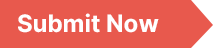### International Journal of Discrete Mathematics

Archive
• 2021, Volume 6

• 2020, Volume 5

• 2019, Volume 4

• 2018, Volume 3

• 2017, Volume 2

• 2016, Volume 1

Submit a Manuscript

Publishing with us to make your research visible to the widest possible audience.Propose a Special Issue

Building a community of authors and readers to discuss the latest research and develop new ideas.### Peaceman Rachford Alternating Direct Implicitly Method for Linear Advection-Diffusion Equation and Its Application

In this paper, Peaceman-Rachford alternating direct implicitly methods presented and applied for solve linear advection-diffusion equation. First, the domain was discretized using the uniform mesh of step length and time step. Secondly, by applying the Taylor series methods, we discretize partial derivative of governing equation and we obtain the central difference equation for Partial differential equation of given governing equation in both duration. Then rearranging the obtained central difference equation; we write the two half scheme of the present method. From each half of these schemes, we obtain tri-diagonal coefficient matrices associated with the system of difference equation. Lastly by applying the Thomas algorithm and writing MATLAB code for the scheme we obtain solution of the governing linear advection diffusion equation. To validate the applicability of the proposed method, three model examples are considered and solved for different values of mesh sizes in both directions. The convergence has been shown in the sense of maximum absolute error (L1-norm) and L2-norm, numerical error and experimental order of convergence. The stability and convergence of the present numerical method are also guaranteed and the comparability of numerical solution and the stability of the present method are presented by using the graphical and tabular form. The numerical results presented in tables and graphs confirm that the approximate solution is in good agreement with the exact solution.

Linear Advection-diffusion Equation, Peaceman-Rachford Alternating Direct Implicitly Method, Taylor Series Methods, Tri-diagonal Coefficient Matrices, Thomas Algorithm, Stability and Convergence

Kedir Aliyi Koroche. (2021). Peaceman Rachford Alternating Direct Implicitly Method for Linear Advection-Diffusion Equation and Its Application. International Journal of Discrete Mathematics, 6(1), 5-14. https://doi.org/10.11648/j.dmath.20210601.12